# Leopoldt conjecture

(diff) ← Older revision | Latest revision (diff) | Newer revision → (diff)

Letbe a totally real algebraic number field (cf. also Field; Algebraic number) and letbe a prime number. Let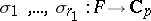denote the distinct embeddings ofinto the completionof the algebraic closure of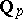. By the Dirichlet unit theorem (cf. also Dirichlet theorem), the unit group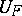ofhas rank. Let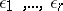be a-basis of. In [a5], H.-W. Leopoldt defined the-adic regulator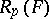as the-adic analogue of the Dirichlet regulator: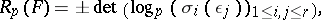where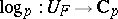denotes the-adic logarithm.

Leopoldt's conjecture is:.

The definition of(and therefore also the conjecture) extends to arbitrary number fields (cf. [a7]) and is nowadays considered in this generality. A. Brumer used transcendental methods developed by A. Baker to prove Leopoldt's conjecture for fieldsthat are Abelian overor over an imaginary quadratic field [a2]. For specific non-Abelian fields the conjecture has also been verified (cf., e.g., [a1]), but in general it is still (1996) open.

For a totally real field, Leopoldt's conjecture is equivalent to the non-vanishing of the-adic-function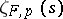at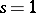(cf. [a5], [a3]).

For a primein, let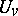denote the group of units of the local field. There is a canonical mapping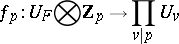and the Leopoldt defectis defined as the-rank of. Class field theory yields the following equivalent formulation of the Leopoldt conjecture (cf. [a7]): Leopoldt's conjecture holds if and only if.

## Relation to Iwasawa theory.

An extension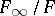of a number fieldis called a-extension if it is a Galois extension and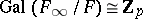. The number of independent-extensions ofis related via class field theory to the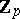-rank of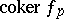and is equal to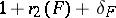(cf. [a4]), whereis the number of pairs of complex-conjugate embeddings of.

For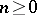, let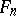denote the unique subfield ofof degreeoverand let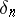denote the Leopoldt defect of. The-extensionsatisfies the weak Leopoldt conjecture if the defectsare bounded independent of. It is known (cf. [a4]) that the weak Leopoldt conjecture holds for the so-called cyclotomic-extension of, i.e. for the unique-extension contained in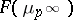.

## Relation to Galois cohomology.

Let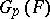denote the Galois group of the maximal pro--extension of, which is unramified outside. Leopoldt's conjecture is equivalent to the vanishing of the Galois cohomology group[a6]. More generally, it is conjectured that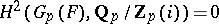for all[a6]. This is known to be true for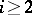as a consequence of a profound result of A. Borel in algebraic-theory.